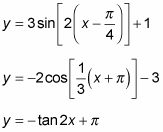##### Trigonometry Workbook For DummiesThe graphs of the trigonometric functions can take on many variations in their shapes and sizes. Starting from the general form, you can apply transformations by changing the amplitude , or the period (interval length), or by shifting the equation up, down, left, or right.

## The general form for a trig function

The general form for the equation of a trigonometry function is y = Af [B (x + C)] + D, where

• f represents the trig function

• A represents the amplitude, or steepness

• +A means the graph is oriented as usual

• A means that the graph is flipped over a horizontal line

• B helps determine the period of the graph (the length of the interval needed for the graph of the function to start repeating itself)

• C determines a shift to the left or right

• D determines a shift up or down

Some examples of trig functions using this format areEach of the numbers changes the basic graph in a particular way.

Here are the ABC’s of reading the general equation for a trig function.

## A is for amplitude in a trigonometry equation

The letter A represents the amplitude of the sine or cosine function, and it affects the steepness or flatness of the graphs of any of the trig functions. If the absolute value (ignore the + or – sign) of A is some number greater than 1, then the graph is steeper than usual. If the absolute value of A is between 0 and 1, then the graph is flatter. The higher the number, the steeper the curve. The closer the number is to 0, the flatter the curve.

## B is for becoming (the period) in a trig equation

The multiplier B affects the length of the graph’s period, or how far it goes along the x-axis. The sine, cosine, cosecant, and secant all normally have a period of 2π. The tangent and cotangent have a period of π. If you divide the normal period of the function by the value of B, then you get the length of the new, adjusted period. Another way to put it: B tells you how many complete cycles the curve will make in the space that usually has only one. If B is 2, then the graph has two complete cycles where there’s usually one.

## C is for cruisin’ left or right in a trigonometry equation

The value of C changes the graph by moving the whole curve to the left or right of where it usually is. If you subtract C, the graph moves C units to the right. If you add C, it moves C units to the left.

## D is for distancing a graph in a trig equation

The value of D tells how far up or down the graph moves from its original position. A positive D moves the graph up, and a negative D moves it down. The value of D also represents the average or middle value of the sine and cosine curves and the middle of the open space of the secant and cosecant curves.